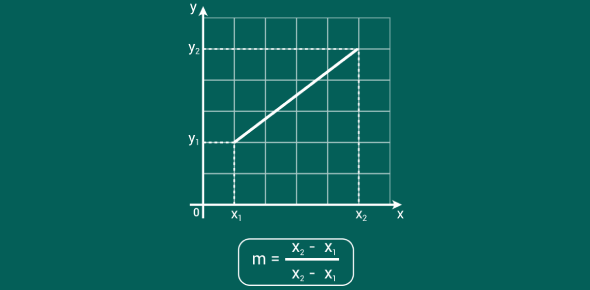# Finding A Slope Given Two Points

Approved & Edited by ProProfs Editorial Team
At ProProfs Quizzes, our dedicated in-house team of experts takes pride in their work. With a sharp eye for detail, they meticulously review each quiz. This ensures that every quiz, taken by over 100 million users, meets our standards of accuracy, clarity, and engagement.
| Written by Vilenatas
V
Vilenatas
Community Contributor
Quizzes Created: 1 | Total Attempts: 9,267
Questions: 7 | Attempts: 9,267SettingsThis quiz is designed to assess if you know how to find a slope given two points on a line. You will also be assessed on steepness of a given slope and horizontal and undefined slopes.

• 1.

### Find the slope of the line passing through the points (2,1), (0,1).

• A.

0

• B.

1

• C.

1/2

• D.

2/1

• E.

-1

A. 0
Explanation
The slope of a line passing through two points can be found using the formula (y2 - y1) / (x2 - x1). In this case, the points are (2,1) and (0,1). Plugging these values into the formula, we get (1 - 1) / (0 - 2) = 0/(-2) = 0. Therefore, the slope of the line passing through these points is 0.

Rate this question:

• 2.

### Find the slope of the line passing through the points (2,5), (2,8).

• A.

1/2

• B.

-3

• C.

-1/2

• D.

2

• E.

Undefined

E. Undefined
Explanation
The slope of a line passing through two points is found using the formula (y2 - y1) / (x2 - x1). In this case, the x-coordinates of both points are the same (2), which means the denominator of the formula would be 0. Division by zero is undefined in mathematics, so the slope of the line passing through these points is also undefined.

Rate this question:

• 3.

### The slope of the line passing through the points (1,4), (-7,4) is ___________.

horizontal
Explanation
The slope of a line is determined by the change in y-coordinates divided by the change in x-coordinates between two points on the line. In this case, both points have the same y-coordinate of 4, indicating that there is no change in the y-coordinate. Therefore, the change in y-coordinates is 0. Since any number divided by 0 is undefined, the slope of the line passing through these points is undefined. In terms of slope types, a line with an undefined slope is considered to be vertical, not horizontal. Therefore, the given answer of "horizontal" is incorrect.

Rate this question:

• 4.

### What is the slope of the line passing through the points (4,2), (8,4)?

• A.

-1/2

• B.

2

• C.

8

• D.

1/2

• E.

-4

D. 1/2
Explanation
The slope of a line passing through two points can be found using the formula (y2-y1)/(x2-x1), where (x1,y1) and (x2,y2) are the coordinates of the two points. In this case, the coordinates are (4,2) and (8,4). Plugging these values into the formula, we get (4-2)/(8-4) = 2/4 = 1/2. Therefore, the slope of the line passing through these points is 1/2.

Rate this question:

• 5.

### Find the slope of the line passing through the points (-6,2), (0,-6).

• A.

-6

• B.

2

• C.

-4/3

• D.

0

• E.

-3/1

C. -4/3
Explanation
The slope of a line passing through two points can be found using the formula: slope = (y2 - y1) / (x2 - x1). In this case, the coordinates of the two points are (-6,2) and (0,-6). Plugging these values into the formula, we get: slope = (-6 - 2) / (0 - (-6)) = -8 / 6 = -4/3. Therefore, the correct answer is -4/3.

Rate this question:

• 6.

### Find the slope of the line passing through the points (-3,-3), (2,2).

• A.

-3

• B.

3

• C.

2

• D.

-2

• E.

1

E. 1
Explanation
The slope of a line passing through two points can be found using the formula (y2 - y1) / (x2 - x1). In this case, the coordinates of the two points are (-3,-3) and (2,2). Plugging these values into the formula, we get (2 - (-3)) / (2 - (-3)) = 5 / 5 = 1. Therefore, the slope of the line passing through these points is 1.

Rate this question:

• 7.

### Find the slope of the line passing through the points (5,-1), (-2,-3).

• A.

2/7

• B.

-5/2

• C.

-1

• D.

-2/7

• E.

-3Back to top